https://doi.org/10.5194/bg-19-4067-2022
https://doi.org/10.5194/bg-19-4067-2022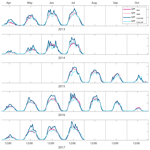# Intercomparison of methods to estimate gross primary production based on CO2 and COS flux measurements

Kukka-Maaria Kohonen, Roderick Dewar, Gianluca Tramontana, Aleksanteri Mauranen, Pasi Kolari, Linda M. J. Kooijmans, Dario Papale, Timo Vesala, and Ivan Mammarella
Abstract

Separating the components of ecosystem-scale carbon exchange is crucial in order to develop better models and future predictions of the terrestrial carbon cycle. However, there are several uncertainties and unknowns related to current photosynthesis estimates. In this study, we evaluate four different methods for estimating photosynthesis at a boreal forest at the ecosystem scale, of which two are based on carbon dioxide (CO2) flux measurements and two on carbonyl sulfide (COS) flux measurements. The CO2-based methods use traditional flux partitioning and artificial neural networks to separate the net CO2 flux into respiration and photosynthesis. The COS-based methods make use of a unique 5-year COS flux data set and involve two different approaches to determine the leaf-scale relative uptake ratio of COS and CO2 (LRU), of which one (LRUCAP) was developed in this study. LRUCAP was based on a previously tested stomatal optimization theory (CAP), while LRUPAR was based on an empirical relation to measured radiation.

For the measurement period 2013–2017, the artificial neural network method gave a GPP estimate very close to that of traditional flux partitioning at all timescales. On average, the COS-based methods gave higher GPP estimates than the CO2-based estimates on daily (23 % and 7 % higher, using LRUPAR and LRUCAP, respectively) and monthly scales (20 % and 3 % higher), as well as a higher cumulative sum over 3 months in all years (on average 25 % and 3 % higher). LRUCAP was higher than LRU estimated from chamber measurements at high radiation, leading to underestimation of midday GPP relative to other GPP methods. In general, however, use of LRUCAP gave closer agreement with CO2-based estimates of GPP than use of LRUPAR. When extended to other sites, LRUCAP may be more robust than LRUPAR because it is based on a physiological model whose parameters can be estimated from simple measurements or obtained from the literature. In contrast, the empirical radiation relation in LRUPAR may be more site-specific. However, this requires further testing at other measurement sites.

Share
Dates
1 Introduction

Photosynthetic carbon uptake (or gross primary production, GPP) is a key component of the global carbon cycle, with the terrestrial ecosystems removing approximately 30 % of annual anthropogenic carbon dioxide (CO2) emissions from the atmosphere . With the current climatic warming it has been suggested that both photosynthesis and respiration are increasing due to the CO2 fertilization effect and rising temperatures providing more favourable conditions not only for photosynthesis but also for respiration . However, it is not known at which rate these two processes are changing and thus the extent to which they offset each other. In addition, their relative importance varies seasonally, with photosynthesis predicted to increase more than respiration in spring, leading to greater carbon uptake, while respiration is predicted to increase more than photosynthesis in autumn, leading to net carbon emission in northern terrestrial ecosystems . Methods to measure and study photosynthesis and respiration individually are thus crucial for future carbon cycle predictions.

Eddy covariance (EC) is widely used to measure the biosphere–atmosphere exchange of CO2 at the ecosystem scale. However, EC only measures net ecosystem CO2 flux (NEE), which includes contributions from both CO2 uptake by photosynthesis (GPP) and ecosystem respiration (R). Traditionally, NEE partitioning into GPP and respiration uses the method of , in which temperature response curves are fitted to nighttime CO2 flux data (respiration). However, this method relies on nighttime EC flux measurements, which are uncertain and often filtered out due to low-turbulence conditions and possible advective gas transport (Aubinet2008). To address this problem, partitioning methods have been developed based on a combination of nighttime temperature responses of respiration (as in nighttime method) and daytime radiation responses of GPP (daytime method) . However, both the nighttime method and the daytime method assume that respiratory processes operate in the same way during the day and night and have uncertainties due to assumptions of functional relationships . These assumptions lead to uncertainties in partitioning because different biomass compartments (soil organic matter, roots, stems, branches, foliage) could have different drivers and respiration responses even within the same ecosystem . Leaf respiration during the day may be inhibited by radiation, the so-called Kok effect , and and suggest that, as a result, global GPP based on the nighttime method has been overestimated. On the other hand, photorespiration, which is an oxidation process competing with carboxylation under radiation, might offset inhibition by the Kok effect .

One way to address these uncertainties in flux partitioning is to use machine learning methods, such as artificial neural networks, to separate NEE into respiration and GPP . The advantage of this method is that it makes no a priori assumptions about responses to environmental drivers but determines these based only on data. In a pioneering study, attempted to use an artificial neural network to emulate the nighttime partitioning method but obtained no significant improvements. More recently, proposed a new approach (NNC-part) involving novel methods for implementing the network's structure and of inferring GPP and R signals from NEE. Both nighttime and daytime NEE are used for network training, so the dynamics of biophysical processes are accounted for in a comprehensive way.

Yet another approach to addressing uncertainties in GPP estimates is to use proxies for photosynthetic CO2 uptake. One such proxy is carbonyl sulfide (COS), which is a sulfur compound with a tropospheric mixing ratio of approximately 500 ppt (parts per trillion) . While the use of different CO2-based partitioning methods is primarily aimed at more accurate GPP estimation, in contrast the use of COS as a proxy for GPP is aimed at a better process understanding of GPP. COS is mainly produced by oceans and anthropogenic sources , while vegetation is the largest sink . COS has been proposed as a proxy for GPP because it is taken up by plants through the same diffusive pathway as CO2 and transported to the chloroplast surface. There it is destroyed by a hydrolysis reaction catalysed by the enzyme carbonic anhydrase (CA, also located within the cytoplasm; ), while CO2 continues its journey inside the chloroplast, where it is assimilated in the Calvin cycle . It is assumed that COS is completely removed by hydrolysis so that there is no back-flux from the leaf to the atmosphere . Estimates of GPP from COS flux measurements use the leaf relative uptake ratio (LRU), that is, the ratio of COS and CO2 deposition rates at the leaf scale. While LRU has been treated either as a global or plant-specific constant , recent studies have shown that LRU is a function of solar radiation because CO2 uptake is highly radiation dependent, while COS uptake is not and may also vary with vapour pressure deficit . In addition to uncertainties related to variation in LRU, COS-based GPP estimates are uncertain because ecosystem-scale COS flux measurements typically have a low signal-to-noise ratio and high random uncertainty at a 30 min timescale, although this is reduced when fluxes are averaged over longer time periods .

In this study, we compare the annual, seasonal, daily, and sub-daily variation of (i) a traditional GPP estimate (GPPNLR, NLR referring to non-linear regression) based on a combination of daytime and nighttime methods, (ii) a neural network GPP estimate based on NEE and NNC-part (GPPANN), (iii) a GPP estimate based on COS flux measurements using the radiation-dependent LRU function from (GPPCOS,PAR), and (iv) a GPP estimate based on COS flux measurements using a previously published stomatal optimization model (CAP) to calculate LRU (GPPCOS,CAP) in a boreal evergreen needle–leaf forest during the years 2013–2017. Our aim is to study potential inconsistencies in diel or seasonal patterns of GPP that may arise from extrapolating nighttime temperature responses to daytime ones, as well as to discuss the limitations and uncertainties of all four methods. We also make recommendations for improving COS-based GPP estimates.

2 Materials and methods

## 2.1 Site description

Measurements were conducted at the Hyytiälä forest Station for Measuring Ecosystem Atmosphere Relations (SMEAR) II measurement site ($\mathrm{61}{}^{\circ }{\mathrm{51}}^{\prime }$ N, $\mathrm{24}{}^{\circ }{\mathrm{17}}^{\prime }$ E), where the forest stand is already more than 50 years old . The stand is dominated by Scots Pine (Pinus Sylvestris L.) with some Norway spruce (Picea abies L. Karst.) and deciduous trees (e.g. Betula sp., Populus tremula, Sorbus aucuparia). The daytime flux footprint covers a ca. 50 ha area of the forest. The canopy height increased from approximately 18 to 20 m during the measurement period (2013–2017), and the all-sided leaf area index (LAI) was ca. 8 m2 m−2.

## 2.2 Measurements

### Eddy covariance fluxes and environmental measurements

EC measurements were made on a 23 m high tower. The set-up consisted of a Gill HS (Gill Instruments Ltd., England, UK) sonic anemometer measuring horizontal and vertical wind velocities and sonic temperature, as well as a quantum cascade laser (QCL; Aerodyne Research Inc., Billerica, MA, USA) for measuring COS, CO2 and H2O mixing ratios at 10 Hz frequency. The set-up is described in more detail in , and flux data are presented in . Flux processing was done using EddyUH software following the methods presented by . Fluxes were corrected for storage change and filtered according to friction velocity. Storage change fluxes of COS were calculated from the COS profile measurements in 2015–2017 and from concentration measurements at one height in other years, as described in ; CO2 storage change fluxes were calculated from CO2 concentration profile measurements. The friction velocity threshold was determined from CO2 fluxes , and a threshold of 0.3 m s−1 was applied to the entire data set to exclude periods of low turbulence. COS flux processing was done similarly to CO2 processing, but time lag and spectral corrections were determined from CO2 measurements and applied to COS as recommended by . Gap-filling of the COS flux was done using empirical formulas based on photosynthetically active radiation (PAR) and vapour pressure deficit (VPD), as described by . CO2 fluxes were gap-filled and partitioned using a procedure to be explained in more detail in Sect. 2.3.1.

Environmental measurements used in the study include air temperature (Ta) at 16.8 m (measured with a Pt100 temperature sensor inside a ventilated custom shield), PAR above the canopy (Li-190SZ quantum sensor, LI-COR, Lincoln, NE, USA), relative humidity (RH) at 16.8 m height (Rotronic MP102H, Rotronic Instrument Corp., NY, USA), soil temperature (Tsoil) at 2–5 cm depth (KTY81-110 temperature sensor, Philips, the Netherlands) as a mean of five locations, and soil water content (SWC) in the humus layer (Delta-T ML2 soil moisture sensor, Delta-T Devices, Cambridge, UK).

## 2.3 GPP calculations

This section describes each of the four methods for estimating GPP. Daily average GPP was only calculated if more than 50 % of the measured 30 min flux data were available for each day, and monthly averages were calculated from the daily means. In , COS fluxes were found to have 52 % data availability on average. While setting a 50 % threshold is somewhat subjective, it ensures that the analysed daily estimates of GPPs reflect measured fluxes rather than the gap-filling procedure. Gap-filled flux data were used in estimating diurnal variation and cumulative GPP. All comparisons between the methods used measured (non-gap-filled) data only, when both CO2 and COS flux data were available.

### 2.3.1 GPP from traditional CO2 flux partitioning

NEE was partitioned into respiration (R) and GPPNLR as

$\begin{array}{}\text{(1)}& \text{NEE}=R-{\text{GPP}}_{\text{NLR}},\end{array}$

where R was estimated as in the nighttime method,

$\begin{array}{}\text{(2)}& R={R}_{\mathrm{C}}{Q}_{\mathrm{10}}^{{T}_{\text{sa}}/\mathrm{10}},\end{array}$

where RC is the respiration at a reference temperature (T=0C), Q10 is the temperature sensitivity of R, and Tsa is the arithmetic mean between the air temperature at 16.8 m height and soil temperature at 5 cm depth. Previous studies have shown Tsa to be a good choice of respiration driver at Hyytiälä forest .

When NEE measurements were not available, the GPP model followed the formula

$\begin{array}{}\text{(3)}& \begin{array}{rl}& {\text{GPP}}_{\text{NLR}}=\\ & \left(\mathit{\alpha }\text{PAR}+{P}_{\text{max}}-\sqrt{\left(\mathit{\alpha }\text{PAR}+{P}_{\text{max}}{\right)}^{\mathrm{2}}-\mathrm{4}\mathrm{\Theta }\text{PAR}{P}_{\text{max}}}\right)\frac{f\left({T}_{\text{a}}\right)}{\mathrm{2}\mathrm{\Theta }}\end{array},\end{array}$

where α, Pmax, and Θ are fitting parameters, and f(Ta) is an instantaneous temperature response that brings GPP gradually towards zero at freezing temperatures, given by

$\begin{array}{}\text{(4)}& f\left({T}_{\mathrm{a}}\right)=\frac{\mathrm{1}}{\mathrm{1}+{e}^{\left(\mathrm{2}\left({T}_{\mathrm{0}}-{T}_{\mathrm{a}}\right)\right)}},\end{array}$

where ${T}_{\mathrm{0}}=-\mathrm{2}$C is the inflection point .

Parameters α, Pmax, and RC were estimated for 15 d periods, while Q10 was estimated from the weighted mean of monthly Q10 values from June to August over several years. Weights were the inverse of the confidence interval of each Q10 estimate. Θ was determined as the value that gave the best model fit when the partitioning was run during summer months (June–August) over several years . The parameters of Eq. (3) were estimated from GPP partitioned with the nighttime method in Eq. (1). The modelled NEE from Eqs. (3) and (2) was compared with the measured NEE in Fig. B1a.

### 2.3.2 GPP from artificial neural networks

GPPANN from the data-driven model was estimated by applying the NNC-part algorithm . NNC-part is a customized neural network that emulates the bio-physical processes driving both GPP and R at ecosystem scale and has been applied to several vegetation types distributed globally. The network consists of two subnetworks, which simulate GPP and R. The two subnetworks are connected in the last node of the overall structure, in which the GPP and R signals are combined to calculate NEE. The GPP subnetwork consists of three layers and estimates the ecosystem-level gross photosynthesis using a light-use efficiency (LUE) approach; in particular, instantaneous LUE is estimated by the first two layers, while GPP is calculated as the product between LUE and incoming shortwave radiation in the third layer. NNC-part has a hybrid nature, and gross photosynthesis is partially constrained by emulating the LUE concept.

Each subnetwork relies on specific predictors. Distinguishing features of this model are that (a) GPP and R derived by other models are not used, (b) functional relationships are derived directly from the data, and (c) the network's weights are tuned by training the machine learning only on NEE measurements. In this experiment we used the same predictors (VPD, incoming shortwave radiation, potential incoming radiation, Ta, Tsoil, SWC, wind speed, and wind direction) and network structure as applied by . However, to ensure the viability of this method, which is limited by the availability of both predictors and NEE measurements, we set lower requirements for the minimum percentage of measured data for both predictors and half hourly NEE. Moreover, data from all available years were pooled for use in a unique multi-year training process. In particular, we applied the following setting: for each year, less than 55 % of predictors were gap-filled, and at least 365 half-hourly NEEs should be measured for both nighttime and daytime. Despite the high percentage of missing data in observations, gaps had generally short duration with limited effects on the uncertainty of predicted outputs. The final GPPANN products were derived by applying trained networks on meteorological inputs and thus do not include NEE data after network training. The modelled NEE from NNC-part was compared with the measured NEE in Fig. B1b.

### 2.3.3 GPP from COS flux measurements and an empirical LRU radiation relation

Based on previous soil chamber measurements at Hyytiälä forest it is known that the soil COS flux was −2.7$\mathrm{pmol}\phantom{\rule{0.125em}{0ex}}{\mathrm{m}}^{-\mathrm{2}}\phantom{\rule{0.125em}{0ex}}{\mathrm{s}}^{-\mathrm{1}}$ on average with a variation of only 1 $\mathrm{pmol}\phantom{\rule{0.125em}{0ex}}{\mathrm{m}}^{-\mathrm{2}}\phantom{\rule{0.125em}{0ex}}{\mathrm{s}}^{-\mathrm{1}}$ during the growing season and a negligible diurnal variation . The average soil flux was thus first subtracted from the quality-filtered and gap-filled COS EC fluxes in order to derive the vegetation contribution to the ecosystem COS exchange. GPP was then calculated from the canopy COS fluxes (FCOS) using the formula

$\begin{array}{}\text{(5)}& {\text{GPP}}_{\text{COS}}=\frac{-\text{FCOS}}{\text{LRU}}\frac{\left[{\text{CO}}_{\mathrm{2}}{\right]}_{\mathrm{a}}}{\left[\text{COS}{\right]}_{\mathrm{a}}},\end{array}$

where [CO2]a and [COS]a denote the atmospheric concentrations of CO2 and COS (in mol m−3), respectively, at the EC measurement height, measured by the QCL.

LRU was calculated as a function of PAR (LRUPAR) as described by the empirical equation of :

$\begin{array}{}\text{(6)}& {\text{LRU}}_{\text{PAR}}=\frac{\mathrm{607.26}}{\text{PAR}}+\mathrm{0.57}.\end{array}$

This LRU equation was derived from field chamber measurements (LRUch) of pine branch CO2 and COS fluxes with two chambers placed at the top of the canopy in 2017 at the same site and thus being independent from the EC flux measurements .

### 2.3.4 GPP from COS flux measurements and LRU from stomatal optimization model

Finally, we estimated GPP from Eq. (5) using a new theoretical expression for LRU (LRUCAP) derived from the stomatal optimization model CAP . Full details of the derivation are given in Appendix A. The LRUCAP formulation was based on the following general expression for LRU given by Eqs. (10)–(11) of :

$\begin{array}{}\text{(7)}& \text{LRU}=\frac{\mathrm{1}}{\mathrm{1}-\frac{{c}_{\mathrm{i}}}{{c}_{\mathrm{a}}}}\frac{\frac{\mathrm{1}}{\mathrm{1.21}}+\frac{\mathrm{1}}{\mathrm{1.14}}\frac{{g}_{\mathrm{s}}^{\text{COS}}}{{g}_{\mathrm{b}}^{\text{COS}}}}{\mathrm{1}+\frac{{g}_{\mathrm{s}}^{\text{COS}}}{{g}_{\mathrm{b}}^{\text{COS}}}+\frac{{g}_{\mathrm{s}}^{\text{COS}}}{{g}_{\mathrm{m}}^{\text{COS}}}},\end{array}$

where ${g}_{x}^{\text{COS}}\left(x=\mathrm{b},\mathrm{s},\mathrm{m}\right)$ are, respectively, the boundary layer, stomatal, and mesophyll conductances for COS, ca is the atmospheric CO2 molar mixing ratio (mol mol−1), ci is the leaf intercellular CO2 molar mixing ratio (mol mol−1), and the numerical factors 1.21 and 1.14 are the ratios of the conductances of CO2 to COS for stomata and the boundary layer . If it is assumed that the boundary layer and mesophyll conductances are infinite (as done by ), Eq. (7) reduces to

$\begin{array}{}\text{(8)}& \text{LRU}=\frac{\mathrm{1}}{\mathrm{1.21}}{\left(\mathrm{1}-\frac{{c}_{\mathrm{i}}}{{c}_{\mathrm{a}}}\right)}^{-\mathrm{1}}.\end{array}$

An analytical expression for ci was derived from the stomatal optimization model CAP by , according to which stomatal conductance maximize leaf photosynthesis, reflecting a trade-off between stomatal limitations to CO2 diffusion and non-stomatal limitations (NSLs) to carboxylation capacity. The CAP model predicts the value of ci as an analytical function of various environmental and physiological factors. Inserting this function into Eq. (8), LRUCAP can then be expressed as

$\begin{array}{}\text{(9)}& {\mathrm{\text{LRU}}}_{\text{CAP}}=\frac{\mathrm{1}}{\mathrm{1.21}}\frac{{c}_{\mathrm{a}}}{{c}_{\mathrm{a}}-{\mathrm{\Gamma }}^{*}}\left(\mathrm{1}+\sqrt{\frac{{K}_{\text{sl}}|{\mathit{\psi }}_{\mathrm{c}}|}{\mathrm{1.6}{g}_{\mathrm{c}}\text{VPD}}}\sqrt{\mathrm{1}+\frac{\mathrm{2}{\mathrm{\Gamma }}^{*}{g}_{\mathrm{c}}}{\mathit{\alpha }\text{PAR}}}\right),\end{array}$

where Γ* is the CO2 photorespiratory compensation point (mol mol−1), Ksl the soil-to-leaf hydraulic conductance ($\mathrm{mol}\phantom{\rule{0.125em}{0ex}}{\mathrm{m}}^{-\mathrm{2}}\phantom{\rule{0.125em}{0ex}}{\mathrm{s}}^{-\mathrm{1}}\phantom{\rule{0.125em}{0ex}}{\mathrm{MPa}}^{-\mathrm{1}}$), ψc is the assumed critical leaf water potential (MPa) at which NSLs reduce photosynthesis to zero, gc is the carboxylation conductance in the absence of NSLs ($\mathrm{mol}\phantom{\rule{0.125em}{0ex}}{\mathrm{m}}^{-\mathrm{2}}\phantom{\rule{0.125em}{0ex}}{\mathrm{s}}^{-\mathrm{1}}$) and α is the photosynthetic quantum yield (mol mol−1) in the absence of NSLs . While Γ*, and α vary seasonally with temperature, for simplicity we used fixed values representing the growing season averages of $\mathrm{50}×{\mathrm{10}}^{-\mathrm{6}}$ and 0.05 mol mol−1, respectively . In addition to PAR ($\mathrm{mol}\phantom{\rule{0.125em}{0ex}}{\mathrm{m}}^{-\mathrm{2}}\phantom{\rule{0.125em}{0ex}}{\mathrm{s}}^{-\mathrm{1}}$) and VPD measurements (mol mol−1), LRUCAP requires soil moisture measurements through its dependence on the soil component of Ksl. All parameter definitions and values are listed in Table 1.

Table 1Explanations, literature values, and sources of the parameters used in the LRUCAP formulation for Hyytiälä forest. ca was derived from the measurements in , and SWC, PAR, and VPD from measurements done in this study. Soil-related values (Ksoil,sat, rcyl, SWCsat, and b) are for soil horizon B (which was considered to be representative of the rooting zone), where the SWC measurements were also made.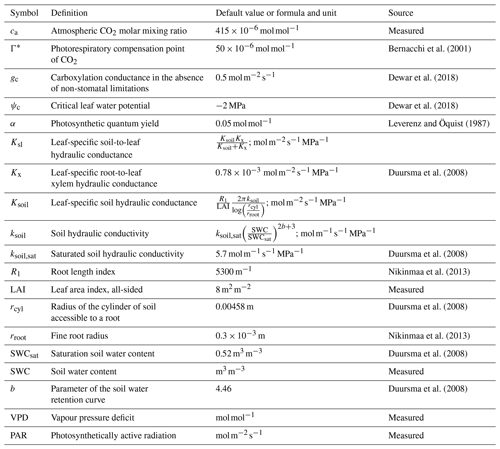LRUCAP is based on a generic physiological model of stomatal function whose predictions have been successfully tested previously (e.g. ). The model parameters are all physiologically meaningful and can be measured independently or obtained from the literature. This formulation therefore represents a clear advance on previous COS-based methods based on empirical fitting (LRUPAR) because it provides a physiological explanation for variations in LRU that may be more robust when extrapolating to other sites.

In addition, LRUCAP was calculated using a combination of literature values and fitted parameters by fitting the parameter combinations $X=|{\mathit{\psi }}_{\mathrm{c}}|/\left(\mathrm{1.6}{g}_{\mathrm{c}}\right)$ (MPa m2 s mol−1) and $Y=\mathrm{2}{\mathrm{\Gamma }}^{*}{g}_{\mathrm{c}}/\mathit{\alpha }$ ($\mathrm{mol}\phantom{\rule{0.125em}{0ex}}{\mathrm{m}}^{-\mathrm{2}}\phantom{\rule{0.125em}{0ex}}{\mathrm{s}}^{-\mathrm{1}}$) to Eq. (9). This analysis was aimed at assessing the parameter sensitivity of LRUCAP. While the literature-based parameter values gave X=2.5 and Y=0.001, the fitting values were X=2.64 and Y=0.0033 and gave a slightly better agreement of LRUCAP with LRUch (RMSE=1.89, while without fitting RMSE=2.01, Fig. B2). However, we emphasize that this fitting procedure was conducted purely in order to assess the model performance and is not a requirement for applying LRUCAP in practice when literature-based parameter values are available. Moreover, the results presented in this article are not based on fitted parameter values but on literature values only.

3 Results and discussion

## 3.1 Environmental conditions

March 2013 was colder than other years (average −7.0C) and also had the highest average PAR (207.3 $\mathrm{µ}\mathrm{mol}\phantom{\rule{0.125em}{0ex}}{\mathrm{m}}^{-\mathrm{2}}\phantom{\rule{0.125em}{0ex}}{\mathrm{s}}^{-\mathrm{1}}$) and lowest soil moisture (0.23 m3 m−3) (Fig. 1). A clear increase in VPD and decrease in soil moisture were seen in August 2013, with soil moisture decreasing from 0.24 in July to 0.19 m3 m−3 in August and afternoon median VPD increasing to 1.00 kPa. July 2014 was warmer (19.0 C) and dryer (VPD 0.88 kPa) than other years, but soil moisture remained high at 0.25 m3 m−3. In 2015, VPD increased from 0.44 in July to 0.62 kPa in August, and soil moisture decreased from 0.31 in July to 0.24 m3 m−3 in August. May 2017 had high amounts of radiation (monthly average PAR of 478.4 $\mathrm{µ}\mathrm{mol}\phantom{\rule{0.125em}{0ex}}{\mathrm{m}}^{-\mathrm{2}}\phantom{\rule{0.125em}{0ex}}{\mathrm{s}}^{-\mathrm{1}}$), and soil temperature was low (3.4 C), while soil moisture and VPD were at a normal level at 0.28 m3 m−3 and 0.47 kPa, respectively. Soil moisture in September–December in 2017 was 10 % higher than other years, while no significant differences between years were found in other environmental variables in late autumn.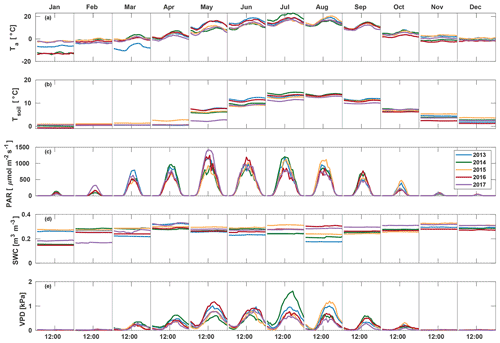Figure 1Median diurnal variation of Ta, Tsoil, PAR, SWC, and VPD in different months during the measurement period 2013–2017.

## 3.2 GPP comparison from sub-daily to seasonal scales

Midday GPPANN was on average 12 % higher than midday GPPNLR during the summer months (May–July) in 2014 and 2017 (Figs. 2, 3, and 4a), opposite to the result found by in a comparison of GPPANN with standard FLUXNET partitioning during summer months at multiple sites. The difference between GPPNLR and GPPANN during other months was negligible. We compared the more common use of air temperature as the respiration driver, GPPairT (instead of the average of soil and air temperatures), against GPPNLR and found that the two methods agreed very well with each other at all timescales (Fig. B3). The small differences in the diurnal variations of GPPNLR and GPPANN are thus not due to the choice of temperature measurement as respiration driver. During the measurement period 2013–2017, 30 min, daily, and monthly GPPANN did not differ statistically from GPPNLR (tested with the ANOVA test; Figs. B4B6). However, on 30 min timescale the GPPANN was on average 15 % lower than GPPNLR. The lower agreement of 30 min GPPANN and GPPNLR than on longer timescales may have resulted from the NNC-part method restricting GPPANN to only positive values, while GPPNLR may take on negative values due to random noise in the NEE measurements. The relative and absolute differences of GPPANN to GPPNLR are, however, very small when averaging over longer time periods (relative difference 2 % on average during summer months, Fig. 5).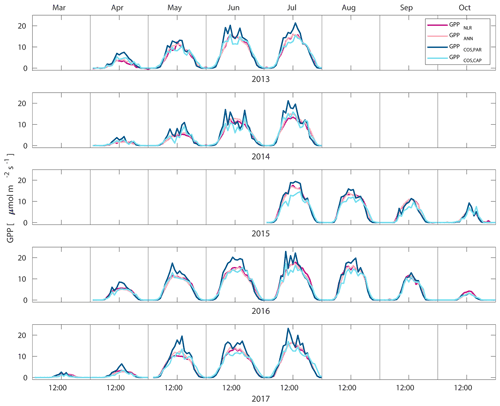Figure 2Median diurnal variation of GPP partitioned using a combined nighttime–daytime method (GPPNLR, purple line), GPP from artificial neural networks (GPPANN, pink line), GPP from COS flux measurements with LRU determined according to (GPPCOS,PAR, dark blue line), and GPP from COS flux measurements using a new approach for LRU (Sect. 2.3.4, GPPCOS,CAP, light blue line) in different months during the measurement period 2013–2017. Averaging was done to the same data points, and only months with more than 55 % of data coverage were included.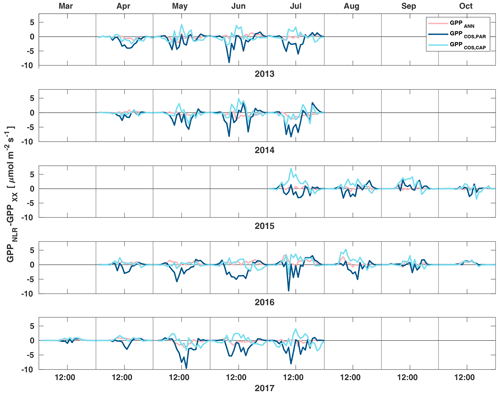Figure 3Diurnal variation of the difference of GPPANN (pink), GPPCOS,PAR (dark blue) and GPPCOS,CAP (light blue) to the reference GPPNLR in different months during the measurement period 2013–2017. Averaging was done to the same data points and only months with more than 55 % of data coverage were included.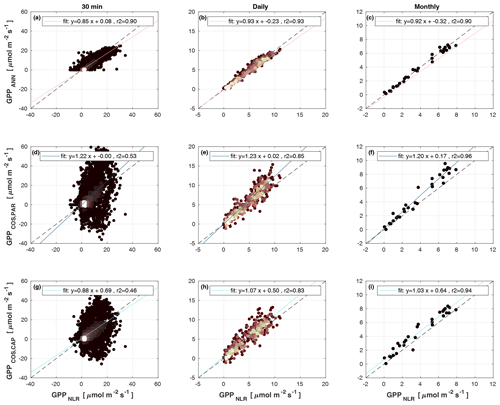Figure 4Scatter plots of GPPANN, GPPCOS,PAR, and GPPCOS,CAP against GPPNLR in 30 min, daily, and monthly timescales. The colour of data points in 30 min and daily scatter plots indicates the data density, lighter colours indicating higher point density than dark ones.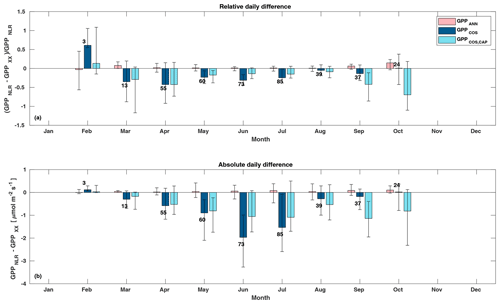Figure 5Relative (a) and absolute (b) difference of daily GPPANN (pink), GPPCOS,PAR (dark blue), and GPPCOS,CAP (light blue) to GPPNLR in different months, averaged over the whole measurement period 2013–2017. Bars represent the median difference, and whiskers show the 25th and 75th percentiles. Numbers on top of the bars indicate how many daily flux data points have been used for calculating the medians. Median differences have been calculated using the same number of data points for each method in each month.

GPPCOS,PAR was very similar to GPPNLR especially during morning and early evening (Figs. 2 and 3) but showed higher midday values than GPPNLR, especially during summer months (May–August) in all years. At the daily scale, GPPCOS,PAR was on average 23 % higher than GPPNLR (Figs. 4e and 5) and also differed from GPPNLR and GPPANN statistically (p<0.01) on 30 min and daily scales (ANOVA test). At monthly scale, there was no statistical difference to any of the other GPP methods.

Based on the CAP stomatal optimization model, LRUCAP requires PAR, SWC, and VPD, as well as ecosystem-specific literature values, for some parameters as input variables. In contrast, LRUPAR by only uses PAR. LRUCAP therefore takes into account additional effects of drought and air humidity on LRU. In spring, the diurnal variation of GPPCOS,CAP closely follows that of GPPNLR and GPPANN until June (Figs. 2 and 3). Especially in June and July GPPCOS,CAP is lower than the other GPP estimates. At 30 min timescale GPPCOS,CAP is on average 12 % lower than GPPNLR, but there is large scatter due to noisy FCOS measurements, like for GPPCOS,PAR. However, there is less scatter in GPPCOS,CAP than GPPCOS,PAR (Fig. 4d and g), indicating that some of the scatter is due to LRU estimation. At daily scales GPPCOS,CAP is 7 % higher than GPPNLR, and at monthly scales the difference decreases to 3 %. However, there is no statistically significant difference between the 30 min and monthly values of GPPNLR and GPPCOS,CAP (ANOVA test). The relative and absolute difference between GPPCOS,CAP and GPPNLR is also generally smaller than between GPPCOS,PAR and GPPNLR throughout the year (Fig. 5). In addition, GPPCOS,CAP reproduces the same two distinctive probability density function peaks as GPPNLR and GPPANN at 1.7 and 6.6 $\mathrm{µ}\mathrm{mol}\phantom{\rule{0.125em}{0ex}}{\mathrm{m}}^{-\mathrm{2}}\phantom{\rule{0.125em}{0ex}}{\mathrm{s}}^{-\mathrm{1}}$, while GPPCOS,PAR finds weaker peaks at 2.4 and 7.4 $\mathrm{µ}\mathrm{mol}\phantom{\rule{0.125em}{0ex}}{\mathrm{m}}^{-\mathrm{2}}\phantom{\rule{0.125em}{0ex}}{\mathrm{s}}^{-\mathrm{1}}$ (Fig. 6). In summary, GPPCOS,CAP gives better agreement with traditional GPPNLR partitioning than GPPCOS,PAR. However, LRUCAP was higher than LRUch and LRUPAR at high radiation (PAR>1000$\mathrm{µ}\mathrm{mol}\phantom{\rule{0.125em}{0ex}}{\mathrm{m}}^{-\mathrm{2}}\phantom{\rule{0.125em}{0ex}}{\mathrm{s}}^{-\mathrm{1}}$, Fig. B7a). This may reflect intrinsic differences in the dependence of LRUPAR and LRUCAP on environmental drivers (PAR, VPD, SWC) as both estimates of LRU are based on conditions at the top of the canopy.

LRUCAP was also calculated based on a combination of literature values and the fitted parameters X and Y (Sects. 2.3.4 and A1) in order to assess the sensitivity to parameter values. While literature values gave X=2.5MPa m2 s mol−1 and Y=0.001$\mathrm{mol}\phantom{\rule{0.125em}{0ex}}{\mathrm{m}}^{-\mathrm{2}}\phantom{\rule{0.125em}{0ex}}{\mathrm{s}}^{-\mathrm{1}}$, fitting gave X=2.64MPa m2 s mol−1 and Y=0.0033$\mathrm{mol}\phantom{\rule{0.125em}{0ex}}{\mathrm{m}}^{-\mathrm{2}}\phantom{\rule{0.125em}{0ex}}{\mathrm{s}}^{-\mathrm{1}}$ and a slightly better agreement of LRUCAP with measured LRU (RMSE=1.89, while without fitting RMSE=2.01). Thus, while X was close to its literature value, Y was estimated to be 3 times higher. This mismatch suggests there may be scope for further model improvement, such as the inclusion of dark respiration and/or finite mesophyll and boundary layer conductances in the LRUCAP model. However, as the difference between fitted LRUCAP and literature-based LRUCAP (statistical significance tested with Student's t test, p<0.01) was not large, with a median difference of 4 %, and the applicability of the model without fitting is better, we decided to use the literature-based parameterization of LRUCAP in this study, without fitting to LRUch.

LRUCAP was also calculated assuming finite mesophyll conductance as a further comparison (Sect. A2). The agreement of this method was better than assuming infinite mesophyll conductance at high PAR but worse at low PAR (Fig. B7d), very similar to the results from , who modelled LRU at Hyytiälä using the ORCHIDEE model. This version of LRUCAP was also fitted to measured LRU in terms of parameters X and Y (Sect. A2) to make the low PAR LRUCAP better, which resulted in X=3.45 and Y=0.0057, both higher than their expected literature values. We thus concluded that the assumption of infinite gm gives an estimate that is closest to LRUch, although the assumption in itself is physiologically unrealistic. found that internal conductance (a combination of mesophyll conductance and biochemical reactions) might limit leaf-scale FCOS during daytime. We find a better agreement of LRUCAP with LRUch if gm is assumed to be infinite, but there is a mismatch at high PAR, supporting the possibility that gm might indeed be a limiting factor under high radiation. In CAP, infinite or finite gm represents two contrasting hypotheses, in which NSLs act either entirely on photosynthetic capacity or entirely on gm, respectively. In reality, NSLs may act on both photosynthetic capacity and gm, with one or the other effect being dominant depending on environmental conditions. The contrasting abilities of each hypothesis to explain LRUch at low vs. high light might be explained by a shift in the action of NSLs from the photosynthetic capacity to gm as light increases. However, verifying this possibility lies beyond the scope of the present study.

We calculated the cumulative GPP estimates over May–July, 13 weeks around the peak growing season for each year (Table 2). Cumulative GPPCOS,PAR was on average 25 % higher than cumulative GPPNLR in all studied years. This is higher than the 4.3 % difference reported in and 3.5 % agreement reported in . In contrast, cumulative GPPCOS,CAP varied from 17 % higher in 2014 to 15 % lower in 2015, and on average it was only 3 % higher than cumulative GPPNLR. Cumulative GPPANN varied from 10 % higher in 2014 to 9 % lower in 2016 than GPPNLR, and on average it was 0.1 % lower than GPPNLR. As stated above, overall GPPANN was closest to GPPNLR out of the three other GPP estimates. GPPCOS,CAP was closer to both of the CO2-based GPP estimates than GPPCOS,PAR. However, at high PAR, LRUCOS,CAP was higher than chamber-based measurements, leading to a lower GPP. Nevertheless, no firm conclusions can be drawn here as the LRU observations only cover measurements at the top of the canopy and may not reflect LRU over the whole canopy.

It has been suggested that, due to the Kok effect, leaf respiration is inhibited under radiation (Kok1949). This inhibition has been estimated to be approximately 13 % in the evergreen needle–leaf forests during summer . Measurements of CO2 isotope fluxes support the conclusion that, due to the Kok effect, GPP from traditional CO2 flux partitioning using the nighttime method is overestimated . However, ecosystem respiration at the Hyytiälä forest site is dominated by soil respiration so that the Kok effect may be of limited importance in this ecosystem (). Reduced leaf respiration under radiation would be visible as a break point around the compensation point with a change in the slope of NEE against radiation. However, such a break point was not detected in our observations, as is demonstrated in Fig. B8. While it is possible that less radiated needles experience less inhibition than well radiated ones that cancel out at the ecosystem scale , this test provides some insight into the problem. It is thus not expected that independent GPP estimates in Hyytiälä would necessarily result in lower GPP than the traditional methods. Moreover, showed that uncertainties and biases in NEE (and COS flux) measurements exceed those resulting from the possible Kok effect.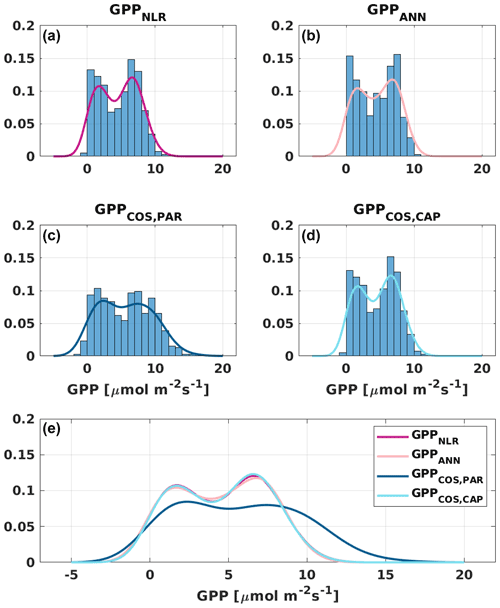Figure 6Distribution (bars) and probability density functions (lines) of daily average (a) GPPNLR, (b) GPPANN, (c) GPPCOS,PAR, and (d) GPPCOS,CAP. All probability density functions are combined in (e) for better comparison.

Table 2Cumulative GPP (g C m−2) over May–July with different GPP estimates. All sums are calculated from the same data coverage, and the fraction of gap-filled flux data (CO2 flux for GPPNLR, COS flux for GPPCOS,PAR and GPPCOS,CAP) is presented in parentheses. GPPANN does not include gap-filled NEE data since it is based on meteorological variables. * In 2015, the cumulative sum covers only July.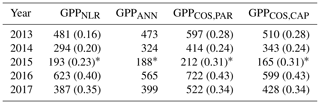## 3.3 GPP responses to environmental conditions

All four GPP estimates responded similarly to environmental forcing (PAR, Ta, VPD) both in spring and summer (Fig. 7). In spring, all GPP estimates increased with increasing radiation levels, while in summer a saturation point was found at PAR>500$\mathrm{µ}\mathrm{mol}\phantom{\rule{0.125em}{0ex}}{\mathrm{m}}^{-\mathrm{2}}\phantom{\rule{0.125em}{0ex}}{\mathrm{s}}^{-\mathrm{1}}$ that could be linked to VPD limitation on stomatal conductance in the afternoon . GPPCOS,PAR was higher than GPPCOS,CAP at PAR>400$\mathrm{µ}\mathrm{mol}\phantom{\rule{0.125em}{0ex}}{\mathrm{m}}^{-\mathrm{2}}\phantom{\rule{0.125em}{0ex}}{\mathrm{s}}^{-\mathrm{1}}$, while at low PAR values they agreed well with each other both in spring and summer, as well as with GPPNLR and GPPANN. GPPCOS,PAR thus has a stronger radiation response than the other GPP estimates due to a lower empirical LRU estimate than LRUCAP at high PAR (Fig. B7). A similar PAR response was found in , who studied GPPCOS,PAR with a traditional GPP partitioning method at four different sites in Europe. Although GPPCOS,CAP agrees well with both GPPNLR and GPPANN at high PAR, it is likely underestimated due to high LRUCAP at high PAR (Fig. B7).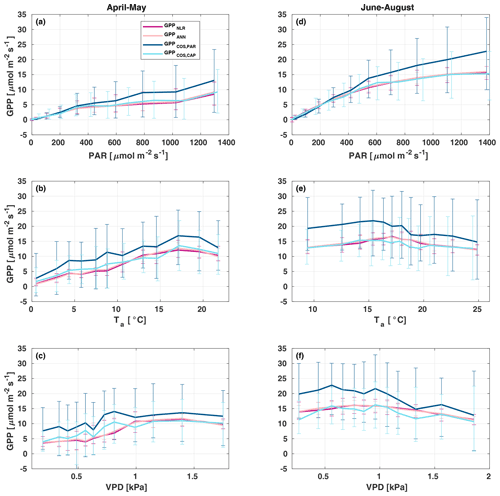Figure 7Responses of the different GPP estimates (GPPNLR (purple), GPPANN (pink), GPPCOS,PAR (dark blue), and GPPCOS,CAP, light blue) to environmental parameters – photosynthetically active radiation (a, d), air temperature (b, e), and vapour pressure deficit (c, f) – in spring (a–c) and summer (d–f). Data are binned to 12 equally sized bins (same number of data points in each bin), and all GPPs have the same data coverage. Only measured (non-gap-filled) 30 min flux data were used, and GPP was filtered to include only PAR>700µmol m2 s−1 in responses to Ta and VPD to avoid simultaneous correlation with PAR.

In spring, increasing air temperature increased all GPP estimates similarly until Ta reached 17 C. However, again GPPCOS,PAR was higher than other GPP estimates. In summer, air temperature did not have a notable effect on any GPP estimate. Responses to VPD were similar for each GPP estimates both in spring and summer. In spring, decreasing air humidity (increasing VPD) was associated with increased GPP until VPD>0.7 kPa, after which VPD had little or no effect. The apparent increase in GPP with VPD in spring may be caused by the correlation of Ta with VPD, coinciding with the start of the growing season as the trees are not water-limited after snowmelt. In summer, dryness started to limit GPP at VPD>1 kPa. We found that similar to PAR and Ta responses, GPPCOS,PAR was higher than other GPP estimates at low VPD values but decreased to similar levels at high VPD (1 kPa) both in spring and summer. GPPCOS,PAR gives higher GPP at low VPD than the CO2-based methods, as does GPPCOS,CAP in spring (Fig. 7). This may indicate that some factor is limiting the photosynthesis reaction (e.g. biochemical limitations in CO2 assimilation) even though the diffusion into the leaf is not limited.

## 3.4 Uncertainties and limitations of the GPP methods

Because ANN fitting is purely based on the provided examples, GPPANN could be more sensitive to the uncertainty of (training) data with respect to the parametric partitioning methods. Moreover, it is sensitive to missing data especially in the case of long data gaps . The method also requires large data sets for training NNC-part, which may not be available at all measurement sites. However, GPPANN does not require prescribed relationships of GPP to environmental data, making it an attractive method for sites with good data availability.

GPPCOS,PAR uses an empirical PAR relation that is based on measurements at Hyytiälä forest. This PAR relation is site-specific and different compared to the one found by . For this reason it is not known if and how it can be used at other sites, where it is suggested to retrieve it directly from observations. The choice of the empirical LRU–PAR relation at any given site is to some extent arbitrary. While the LRUPAR function is simple and thereby attractive, it does not take into account the different light conditions inside the canopy, stomatal regulation during drought, or the effects of non-stomatal limitations on photosynthesis. Moreover, being an empirical model, it does not provide a process-based understanding for LRU. While the results of GPPCOS,PAR are promising, we found a 25 % difference in midday GPP during summer, similar to that found by . We did not find as good an agreement with CO2-based GPP estimates as , who found an agreement within 15 % using a constant LRU of 1.6 in Mediterranean pine forests and crop fields. However, they also reported higher GPPCOS assumed to be related to soil COS uptake, which was not measured or taken into account in their GPP calculations. In our study, we subtracted an average measured soil flux from the ecosystem COS uptake. As the diurnal variation in soil COS exchange was small (less than 1 $\mathrm{pmol}\phantom{\rule{0.125em}{0ex}}{\mathrm{m}}^{-\mathrm{2}}\phantom{\rule{0.125em}{0ex}}{\mathrm{s}}^{-\mathrm{1}}$) throughout the season, averaging did not make a large difference, and thus soil does not explain the differences found here. However, as soil COS flux measurements are not necessarily available at all sites, this may be one source of uncertainty in wider applications. studied COS flux components and GPPCOS in a Mediterranean citrus orchard and found GPPCOS to be on average 7 % lower than traditionally partitioned GPP. They also presented a light-dependent and seasonally varying LRU which, however, could not be applied to Hyytiälä COS fluxes due to the very different ecosystem types studied, indicating that the PAR responses may differ between ecosystems.

GPPCOS,CAP may be more applicable at other sites than GPPCOS,PAR because it is based on a generic physiological model of stomatal behaviour, which requires only literature-based parameter values and simple meteorological variables as inputs. However, as for LRUPAR, LRUCAP should also be tested at other sites against measured LRU to verify its applicability to other ecosystems. The version of LRUCAP assuming infinite mesophyll conductance, while giving reasonable results in comparison with LRUch, is clearly physiologically unrealistic. The formulation of LRUCAP with finite gm did not compare as well with LRUch at Hyytiälä forest (RMSE=2.58, median difference to LRUch 22 %), especially during low light conditions, but may compare better at other measurement sites.

One source of uncertainty in GPP estimates based on LRUCAP and LRUPAR is that both LRU predictions are calculated from radiation measurements at the top of the canopy, where there is no shading by foliage, although the theoretical dependence of LRUCAP on radiation is more generally applicable throughout the canopy. The branch chamber measurements (on which the empirical LRUPAR function is based) were also made at the top of the canopy. The measured needles were thus well-adjusted to high radiation conditions. Therefore, we did not take into account light penetration and scattering through the canopy. However, the needles and leaves within the canopy are also well adjusted to low light conditions and may be more efficient with their stomatal control in varying light conditions than needles on top of the canopy. Thus, this may not be a large source of uncertainty. However, it is also possible that LRU varies throughout the canopy due to different light conditions.

4 Conclusions

Daily GPPANN and GPPNLR did not differ significantly, and differences were also small at sub-daily and seasonal timescales. GPPCOS,PAR was higher than GPPNLR at all timescales studied, including the estimate of 3-month cumulative GPP during the peak growing season. In contrast, GPPCOS,CAP, a new method based on stomatal optimization theory, gave better agreement with GPPNLR at all timescales and was also less scattered than GPPCOS,PAR at a 30 min timescale.

The LRUCAP function provides a new theoretical underpinning for COS-based GPP estimates that can be used at other measurement sites, potentially without requiring additional branch chamber measurements. LRUCAP represents a significant improvement on previous LRU functions based on site-specific empirical regressions. However, LRUCAP overestimated LRU at high radiation when compared to LRU observations at the top of the canopy, leading to a lower midday GPPCOS,CAP, especially in summer. This discrepancy may result from the assumption of infinite mesophyll conductance, or the absence of dark respiration, in the underlying stomatal optimization model. LRUCAP would benefit from further testing at other measurement sites with COS and CO2 branch flux measurements, including measurements inside the canopy for better canopy-integrated LRU estimates.

Although COS flux measurements are noisier, more expensive, and more difficult than those of CO2, they provide an opportunity for better process-based understanding of photosynthesis in comparison to more traditional CO2-based estimates of GPP. In addition to COS, other proxies such as solar-induced fluorescence and isotopic flux measurements should be tested simultaneously to properly investigate their deficiencies and advantages in estimating GPP and processes underlying photosynthesis.

The establishment of large long-term ecosystem research infrastructures (e.g. ICOS, NEON, TERN; see ) – involving sites equipped with eddy covariance systems that could potentially also host COS, SIF (solar-induced fluorescence), and isotope sensors – together with the planned launch of the FLEX satellite in 2025 (https://earth.esa.int/eogateway/missions/flex, last access: 28 June 2022) that will provide global vegetation fluorescence measurements, opens up a new phase in monitoring and understanding plant photosynthesis. Our results also underline the important role of small-scale ecophysiological measurements and models in underpinning these larger-scale initiatives.

Appendix A: LRU predicted by the CAP stomatal optimization model

## A1 LRUCAP assuming infinite mesophyll conductance

The general expression for the leaf relative uptake ratio (LRU) derived from the diffusion laws for COS and CO2 is

$\begin{array}{}\text{(A1)}& \text{LRU}=\frac{\mathrm{1}}{\mathrm{1}-\frac{{c}_{\mathrm{i}}}{{c}_{\mathrm{a}}}}\frac{\frac{\mathrm{1}}{\mathrm{1.21}}+\frac{\mathrm{1}}{\mathrm{1.14}}\frac{{g}_{\mathrm{s}}^{\text{COS}}}{{g}_{\mathrm{b}}^{\text{COS}}}}{\mathrm{1}+\frac{{g}_{\mathrm{s}}^{\text{COS}}}{{g}_{\mathrm{b}}^{\text{COS}}}+\frac{{g}_{\mathrm{s}}^{\text{COS}}}{{g}_{\mathrm{m}}^{\text{COS}}}},\end{array}$

where ${g}_{x}^{\text{COS}}\left(x=\mathrm{b},\mathrm{s},\mathrm{m}\right)$ are the boundary layer, stomatal and mesophyll conductances for COS, respectively, ca and ci are the atmospheric and leaf intercellular CO2 molar mixing ratios (mol mol−1), respectively, and the numerical factors 1.21 and 1.14 are the ratios of the conductances of CO2 to COS for stomata and the boundary layer, respectively.

If it is assumed that boundary layer and mesophyll conductances are infinite, Eq. (A1) reduces to

$\begin{array}{}\text{(A2)}& \text{LRU}=\frac{\mathrm{1}}{\mathrm{1.21}}{\left(\mathrm{1}-\frac{{c}_{\mathrm{i}}}{{c}_{\mathrm{a}}}\right)}^{-\mathrm{1}}.\end{array}$

We derived ci from the CAP stomatal optimization model , according to which stomatal conductance adjusts to maximize the rate of leaf photosynthesis (A) through a trade-off between stomatal and non-stomatal limitations. Our photosynthesis model is based on that of (their Eq. 9.12i), modified to include non-stomatal limitations (NSLs):

$\begin{array}{}\text{(A3)}& A=\left(\mathrm{1}-\frac{{\mathit{\psi }}_{\text{leaf}}}{{\mathit{\psi }}_{\mathrm{c}}}\right)\frac{\mathit{\alpha }Q{g}_{\mathrm{c}}\left({c}_{\mathrm{i}}-{\mathrm{\Gamma }}^{*}\right)}{\mathit{\alpha }Q+{g}_{\mathrm{c}}\left({c}_{\mathrm{i}}+{\mathrm{\Gamma }}^{*}\right)},\end{array}$

where α is the photosynthetic quantum yield (mol mol−1) in the absence of NSLs, Q ($\mathrm{mol}\phantom{\rule{0.125em}{0ex}}{\mathrm{m}}^{-\mathrm{2}}\phantom{\rule{0.125em}{0ex}}{\mathrm{s}}^{-\mathrm{1}}$) is photosynthetically active radiation (PAR), gc ($\mathrm{mol}\phantom{\rule{0.125em}{0ex}}{\mathrm{m}}^{-\mathrm{2}}\phantom{\rule{0.125em}{0ex}}{\mathrm{s}}^{-\mathrm{1}}$) is the initial slope of the Aci response curve in the absence of NSLs, Γ* (mol mol−1) is the photorespiratory CO2 compensation point, ψleaf (MPa) is the leaf water potential, and ψc (MPa) is the critical leaf water potential at which NSLs reduce photosynthesis to zero. In Eq. (A3), NSLs are represented as an apparent downregulation of the Aci response curve by a factor that decreases with decreasing leaf water potential, as has been observed in numerous experiments (e.g. Lintunen et al.2020; Salmon et al.2020). Consequently, as stomatal conductance increases there is a trade-off between increased CO2 supply and increased NSLs such that A has a maximum at some optimal value of stomatal conductance.

We used Eq. (A3) rather than the Farquhar photosynthesis model because, in the latter, the abrupt switch from RuBisCo to electron transport limitation introduces artificial discontinuities in the CAP solution for optimal stomatal conductance , whereas in Eq. (A3) there is a smooth transition from CO2 limitation to light limitation, and no such discontinuities occur. The parameter gc is equivalent to ${V}_{\text{cmax}}/\left({k}_{\mathrm{m}}+{\mathrm{\Gamma }}^{*}\right)$ in the Farquhar model.

The CAP solution for optimal stomatal conductance predicts that

$\begin{array}{}\text{(A4)}& \frac{{c}_{\mathrm{i}}-{\mathrm{\Gamma }}^{*}}{{c}_{\mathrm{a}}-{\mathrm{\Gamma }}^{*}}=\frac{\mathrm{1}}{\mathrm{1}+\mathit{\beta }},\end{array}$

where

$\begin{array}{}\text{(A5)}& \mathit{\beta }=\sqrt{\frac{\mathrm{1.6}D}{{K}_{\text{sl}}|{\mathit{\psi }}_{\mathrm{c}}|}{\left(\frac{\mathrm{1}}{{g}_{\mathrm{c}}}+\frac{\mathrm{2}{\mathrm{\Gamma }}^{*}}{\mathit{\alpha }Q}\right)}^{-\mathrm{1}}},\end{array}$

in which D is vapour pressure deficit (VPD; mol mol−1), and Ksl is the leaf-specific soil-to-leaf hydraulic conductance ($\mathrm{mol}\phantom{\rule{0.125em}{0ex}}{\mathrm{m}}^{-\mathrm{2}}\phantom{\rule{0.125em}{0ex}}{\mathrm{s}}^{-\mathrm{1}}\phantom{\rule{0.125em}{0ex}}{\mathrm{MPa}}^{-\mathrm{1}}$). Writing Eq. (A2) in the equivalent form

$\begin{array}{}\text{(A6)}& \text{LRU}=\frac{\mathrm{1}}{\mathrm{1.21}}\frac{{c}_{\mathrm{a}}}{{c}_{\mathrm{a}}-{\mathrm{\Gamma }}^{*}}{\left(\mathrm{1}-\frac{{c}_{\mathrm{i}}-{\mathrm{\Gamma }}^{*}}{{c}_{\mathrm{a}}-{\mathrm{\Gamma }}^{*}}\right)}^{-\mathrm{1}}\end{array}$

and substituting the CAP prediction from Eqs. (A4) and (A5) then give

$\begin{array}{}\text{(A7)}& \begin{array}{rl}& {\text{LRU}}_{\text{CAP}}=\\ & \frac{\mathrm{1}}{\mathrm{1.21}}\frac{{c}_{\mathrm{a}}}{{c}_{\mathrm{a}}-{\mathrm{\Gamma }}^{*}}\left(\mathrm{1}+\sqrt{\frac{{K}_{\text{sl}}|{\mathit{\psi }}_{\mathrm{c}}|}{\mathrm{1.6}D{g}_{\mathrm{c}}}}\sqrt{\mathrm{1}+\frac{\mathrm{2}{\mathrm{\Gamma }}^{*}{g}_{\mathrm{c}}}{\mathit{\alpha }Q}}\right).\end{array}\end{array}$

In Eq. (A7) all the parameters are physiologically meaningful and can be measured independently or obtained from the literature because the underlying CAP model is based entirely on such parameters. This contrasts with the use of the stomatal optimization model of , for example, which contains an undetermined parameter (λ, interpreted as the marginal water cost of carbon gain) that must be empirically fitted.

Nevertheless, to assess the performance of LRUCAP obtained from literature-based parameter values, we compared it with LRUCAP obtained by fitting the two key parameter combinations $X=|{\mathit{\psi }}_{\mathrm{c}}|/\left(\mathrm{1.6}{g}_{\mathrm{c}}\right)$ and $Y=\mathrm{2}{\mathrm{\Gamma }}^{*}{g}_{\mathrm{c}}/\mathit{\alpha }$ , in terms of which Eq. (A7) may be written as

$\begin{array}{}\text{(A8)}& {\text{LRU}}_{\text{CAP}}=\frac{\mathrm{1}}{\mathrm{1.21}}\frac{{c}_{\mathrm{a}}}{{c}_{\mathrm{a}}-{\mathrm{\Gamma }}^{*}}\left(\mathrm{1}+\sqrt{\frac{{K}_{\text{sl}}X}{D}}\sqrt{\mathrm{1}+\frac{Y}{Q}}\right).\end{array}$

Parameters X and Y were optimized to minimize the RMSE of log (LRUCAP) to measured log (LRU), due to the logarithmic nature of LRU, with MATLAB's fminsearch function. However, we emphasize that this fitting procedure was conducted purely in order to assess the model performance and is not a requirement for applying LRUCAP in practice when literature-based parameter values are available. Moreover, the results presented in this study are not based on the optimized values but on literature values only.

## A2 LRUCAP assuming finite mesophyll conductance

In the case that mesophyll conductance is not assumed to be infinite (but boundary layer conductance is infinite), Eq. (A1) becomes

$\begin{array}{}\text{(A9)}& \text{LRU}=\frac{\mathrm{1}}{\mathrm{1.21}}\frac{\mathrm{1}}{\mathrm{1}+\frac{{g}_{\mathrm{s}}^{\text{COS}}}{{g}_{\mathrm{m}}^{\text{COS}}}}{\left(\mathrm{1}-\frac{{c}_{\mathrm{i}}}{{c}_{\mathrm{a}}}\right)}^{-\mathrm{1}}.\end{array}$

If we further assume that the ratios of stomatal to mesophyll conductances are the same for CO2 and COS, then from ${g}_{\mathrm{s}}^{{\mathrm{CO}}_{\mathrm{2}}}\left({c}_{\mathrm{a}}-{c}_{\mathrm{i}}\right)={g}_{\mathrm{m}}^{{\mathrm{CO}}_{\mathrm{2}}}\left({c}_{\mathrm{i}}-{c}_{\mathrm{c}}\right)$, where cc is the chloroplast CO2 molar mixing ratio (mol mol−1), we can make the substitution

$\begin{array}{}\text{(A10)}& \frac{{g}_{\mathrm{s}}^{\text{COS}}}{{g}_{\mathrm{m}}^{\text{COS}}}=\frac{{g}_{\mathrm{s}}^{{\mathrm{CO}}_{\mathrm{2}}}}{{g}_{\mathrm{m}}^{{\mathrm{CO}}_{\mathrm{2}}}}=\frac{{c}_{\mathrm{i}}-{c}_{\mathrm{c}}}{{c}_{\mathrm{a}}-{c}_{\mathrm{i}}}\end{array}$

in Eq. (A9) to obtain

$\begin{array}{}\text{(A11)}& \text{LRU}=\frac{\mathrm{1}}{\mathrm{1.21}}{\left(\mathrm{1}-\frac{{c}_{\mathrm{c}}}{{c}_{\mathrm{a}}}\right)}^{-\mathrm{1}},\end{array}$

which reduces to Eq. (A2) when mesophyll conductance is infinite (since then cc=ci). As noted above, CAP represents NSLs in terms of an apparent downregulation of the Aci response curve (Eq. A3). This empirical observation may be interpreted in various ways: as a downregulation of photosynthetic efficiencies (α and gc) in the chloroplast, a downregulation of mesophyll conductance (${g}_{\mathrm{m}}^{{\mathrm{CO}}_{\mathrm{2}}}$), or some combination of the two. In the case when NSLs act entirely on ${g}_{\mathrm{m}}^{\text{COS}}$ with no effect on the biochemical efficiencies, A is given as a function of the chloroplast CO2 concentration by (cf. Eq. A3)

$\begin{array}{}\text{(A12)}& A=\frac{\mathit{\alpha }Q{g}_{\mathrm{c}}\left({c}_{\mathrm{c}}-{\mathrm{\Gamma }}^{*}\right)}{\mathit{\alpha }Q+{g}_{\mathrm{c}}\left({c}_{\mathrm{c}}+{\mathrm{\Gamma }}^{*}\right)}.\end{array}$

In this case, since Eq. (A3) still holds, we obtain the same optimal CAP solution for stomatal conductance and ci (Eq. A4) as before but now with an additional prediction for the finite (but variable) mesophyll conductance as implied by Eq. (A12), which links the chloroplast CO2 concentration (cc) to the CAP solution of A. From Eq. (A12),

$\begin{array}{}\text{(A13)}& {c}_{\mathrm{c}}-{\mathrm{\Gamma }}^{*}=\frac{\left(\frac{\mathit{\alpha }Q}{{g}_{\mathrm{c}}}+\mathrm{2}{\mathrm{\Gamma }}^{*}\right)A}{\mathit{\alpha }Q-A}.\end{array}$

The CAP solution for stomatal conductance is given by

$\begin{array}{}\text{(A14)}& {g}_{\mathrm{s}}=\frac{\mathit{\alpha }Q}{\frac{\mathit{\alpha }Q}{{g}_{\mathrm{c}}}+\mathrm{2}{\mathrm{\Gamma }}^{*}}\frac{x\mathit{\theta }}{x{\mathit{\beta }}^{\mathrm{2}}+\left(\mathrm{1}-x\right)\left(xw+\mathrm{1}\right)},\end{array}$

where

$\begin{array}{}\text{(A15)}& \mathit{\theta }& =\mathrm{1}-\frac{{\mathit{\psi }}_{\text{soil}}}{{\mathit{\psi }}_{\mathrm{c}}},\text{(A16)}& w& =\frac{{c}_{\mathrm{a}}-{\mathrm{\Gamma }}^{*}}{\frac{\mathit{\alpha }Q}{{g}_{\mathrm{c}}}+\mathrm{2}{\mathrm{\Gamma }}^{*}},\text{(A17)}& x& =\frac{{c}_{\mathrm{i}}-{\mathrm{\Gamma }}^{*}}{{c}_{\mathrm{a}}-{\mathrm{\Gamma }}^{*}}=\frac{\mathrm{1}}{\mathrm{1}+\mathit{\beta }},\end{array}$

in which ψsoil (MPa) is the soil water potential. Substituting x as a function of β into Eq. (A14) and simplifying give

$\begin{array}{}\text{(A18)}& {g}_{\mathrm{s}}=\frac{\mathit{\alpha }Q}{\frac{\mathit{\alpha }Q}{{g}_{\mathrm{c}}}+\mathrm{2}{\mathrm{\Gamma }}^{*}}\frac{\mathit{\theta }}{\mathit{\beta }\left(\mathrm{1}+\mathit{\beta }+\frac{w}{\mathrm{1}+\mathit{\beta }}\right)}.\end{array}$

We then find the CAP solution for A as follows:

$\begin{array}{}\text{(A19)}& \begin{array}{rl}A& ={g}_{\mathrm{s}}\left({c}_{\mathrm{a}}-{c}_{\mathrm{i}}\right)\\ & ={g}_{\mathrm{s}}\left({c}_{\mathrm{a}}-{\mathrm{\Gamma }}^{*}\right)\left(\mathrm{1}-x\right)\\ & ={g}_{\mathrm{s}}\left({c}_{\mathrm{a}}-{\mathrm{\Gamma }}^{*}\right)\frac{\mathit{\beta }}{\mathrm{1}+\mathit{\beta }}\\ & =\frac{\mathit{\alpha }Q\left({c}_{\mathrm{a}}-{\mathrm{\Gamma }}^{*}\right)}{\frac{\mathit{\alpha }Q}{{g}_{\mathrm{c}}}+\mathrm{2}{\mathrm{\Gamma }}^{*}}\frac{\mathit{\theta }}{\left(\mathrm{1}+\mathit{\beta }{\right)}^{\mathrm{2}}+w}.\end{array}\end{array}$

Substituting this into Eq. (A13) and simplifying then give

$\begin{array}{}\text{(A20)}& \begin{array}{rl}\frac{{c}_{\mathrm{c}}-{\mathrm{\Gamma }}^{*}}{{c}_{\mathrm{a}}-{\mathrm{\Gamma }}^{*}}& =\frac{\mathit{\theta }}{\left(\mathrm{1}+\mathit{\beta }{\right)}^{\mathrm{2}}+\left(\mathrm{1}-\mathit{\theta }\right)w}\\ & =\frac{\mathit{\theta }}{\left(\mathrm{1}+\mathit{\beta }{\right)}^{\mathrm{2}}+\left(\mathrm{1}-\mathit{\theta }\right)\frac{{g}_{\mathrm{c}}\left({c}_{\mathrm{a}}-{\mathrm{\Gamma }}^{*}\right)}{\mathit{\alpha }Q+\mathrm{2}{\mathrm{\Gamma }}^{*}{g}_{\mathrm{c}}}},\end{array}\end{array}$

which can be combined with Eq. (A11) to give the solution of LRUCAP with finite mesophyll conductance.

As for LRUCAP with infinite mesophyll conductance, we also fitted this version with respect to parameters X and Y in order to compare it with the performance of the model using literature-based values. For this procedure, β and w were expressed in terms of X and Y,

$\begin{array}{}\text{(A21)}& \mathit{\beta }=\frac{\mathrm{1}}{\sqrt{\frac{{K}_{\text{sl}}X}{D}\left(\mathrm{1}+\frac{Y}{Q}\right)}}\end{array}$

and

$\begin{array}{}\text{(A22)}& w=\frac{{c}_{\mathrm{a}}-{\mathrm{\Gamma }}^{*}}{\mathrm{2}{\mathrm{\Gamma }}^{*}}\frac{\mathrm{1}}{\frac{Q}{Y}+\mathrm{1}},\end{array}$

and then substituted into Eq. (A20). However, as for the infinite gm solution, this fitting procedure was conducted purely in order to assess the model performance and is not a requirement for applying LRUCAP in practice when literature-based parameter values are available.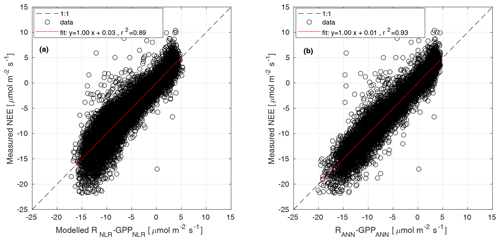Figure B1Modelled against measured NEE using (a) NLR and (b) ANN models for modelling NEE.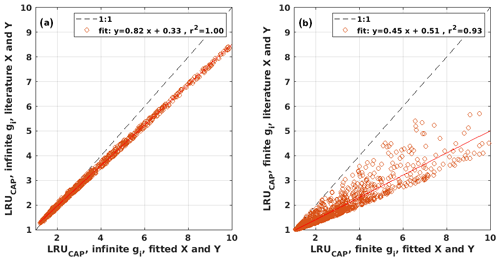Figure B2Scatter plots of LRUCAP using the literature values against LRUCAP using the optimized parameter values when assuming (a) infinite or (b) finite mesophyll conductance.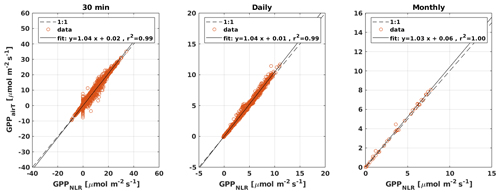Figure B3Scatter plots of GPPairT that uses only air temperature as the driver for respiration against GPPNLR that uses an average of air and soil temperatures as the respiration driver at 30 min, daily, and monthly timescales. The solid black line is the least-squares linear fit to the data.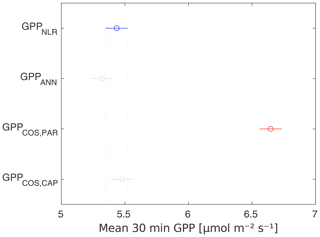Figure B4ANOVA test results for 30 min GPP data. Gray bars indicate no difference to the reference (blue), and red bars indicate statistical difference to the reference. The results show that only GPPCOS,PAR differs statistically from GPPNLR at 30 min timescale.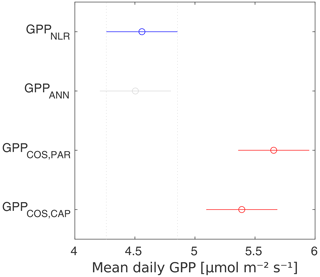Figure B5ANOVA test results for daily GPP data. Gray bars indicate no difference to the reference (blue), and red bars indicate statistical difference to the reference. The results show that both GPPCOS,PAR and GPPCOS,CAP differ statistically from both GPPNLR and GPPANN at daily scale. GPPCOS,PAR and GPPCOS,CAP do not differ from each other.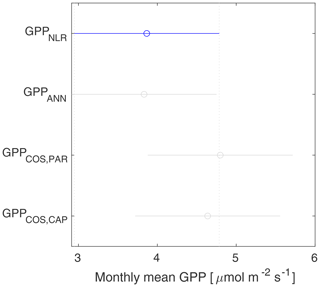Figure B6ANOVA test results for monthly GPP data. Gray bars indicate no difference to the reference (blue), and red bars indicate statistical difference to the reference. The results show that all GPPs are statistically the same at monthly scale.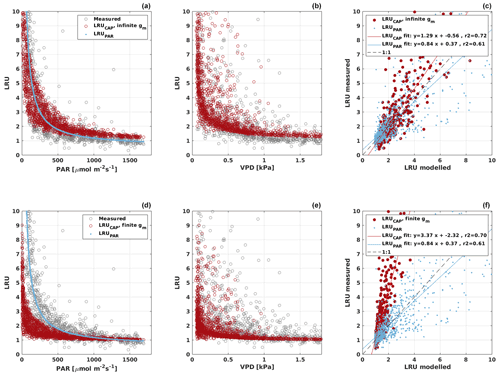Figure B7LRU derived from chamber measurements (gray) and modelled LRUPAR (blue) and LRUCAP (red) assuming infinite (a–c) or finite (d–f) mesophyll conductance (gm) in LRUCAP against PAR and VPD. Subplots (c) and (d) compare the chamber-measured LRU against modelled LRUPAR and LRUCAP.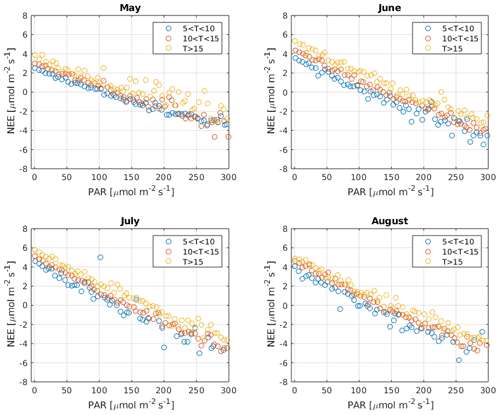Figure B8Net ecosystem exchange (NEE) against photosynthetically active radiation (PAR) close to the compensation point during May, June, July, and August. Data are binned to different air temperature classes: $\mathrm{5}\phantom{\rule{0.125em}{0ex}}{}^{\circ }\mathrm{C}<{T}_{\mathrm{a}}<\mathrm{10}$C (blue), $\mathrm{10}\phantom{\rule{0.125em}{0ex}}{}^{\circ }\mathrm{C}<{T}_{\mathrm{a}}<\mathrm{15}$C (orange), and Ta>15C (yellow).

Data availability

The flux data and all GPP estimates used in this study are available from https://doi.org/10.5281/zenodo.6940750 . Environmental data used in the study are available from http://urn.fi/urn:nbn:fi:att:a8e81c0e-2838-4df4-9589-74a4240138f8 . The most recent version of the data is available from https://smear.avaa.csc.fi (last access: 9 June 2020).

Author contributions

KMK, IM, and TV designed the study. KMK, PK, and LMJK performed the measurements and flux processing. RD, AM, and KMK developed the new LRU formulation. GT provided the GPP estimate by artificial neural networks. DP gave insight into all GPP method uncertainties and study design and commented on the manuscript. All authors contributed by commenting on the study design, results, and the manuscript. KMK wrote the manuscript with contributions from all co-authors.

Competing interests

The contact author has declared that none of the authors has any competing interests.

Disclaimer

Publisher's note: Copernicus Publications remains neutral with regard to jurisdictional claims in published maps and institutional affiliations.

Acknowledgements

Special thanks to Helmi Keskinen, Sirpa Rantanen, Janne Levula, and other Hyytiälä technical staff for all their support with the measurements.

Financial support

This research has been supported by the Academy of Finland (grant nos. 118780, 312571, 282842, 337549, and 342930), the H2020 European Research Council (grant nos. 755617 and 742798), the H2020 Environment (grant no. 820852), and ICOS Finland (grant no. 3119871). Specifically, Gianluca Tramontana was supported by the European Research Council (ERC) under the ERC-2017-STG SENTIFLEX project (grant no. 755617), Linda M. J. Kooijmans was supported by the ERC advanced funding scheme (AdG 2016, grant number 742798, project abbreviation COS-OCS), and Dario Papale was supported by the E-SHAPE H2020 project (grant no. 820852) and the ICOS-ETC. Kukka-Maaria Kohonen was supported by the Vilho, Yrjö, and Kalle Väisälä foundation. Aleksanteri Mauranen was supported by the Doctoral Programme in Atmospheric Sciences of the University of Helsinki.

Open-access funding was provided by the Helsinki University Library.

Review statement

This paper was edited by Nicolas Brüggemann and reviewed by three anonymous referees.

References

Aalto, J., Aalto, P., Keronen, P., Kolari, P., Rantala, P., Taipale, R., Kajos, M., Patokoski, J., Rinne, J., Ruuskanen, T., Leskinen, M., Laakso, H., Levula, J., Pohja, T., Siivola, E., and Kulmala, M.: SMEAR II Hyytiälä forest meteorology, greenhouse gases, air quality and soil (Version 1), University of Helsinki, Institute for Atmospheric and Earth System Research [data set], http://urn.fi/urn:nbn:fi:att:a8e81c0e-2838-4df4-9589-74a4240138f8 (last access: 9 June 2020), 2019. a

Asaf, D., Rotenberg, E., Tatarinov, F., Dicken, U., Montzka, S. A., and Yakir, D.: Ecosystem photosynthesis inferred from measurements of carbonyl sulphide flux, Nat. Geosci., 6, 186–190, https://doi.org/10.1038/NGEO1730, 2013. a, b

Aubinet, M.: Eddy covariance CO2 flux measurements in nocturnal conditions: an analysis of the problem, Ecol. Appl., 18, 1368–1378, 2008. a

Bernacchi, C., Singsaas, E., Pimentel, C., Portis Jr., A., and Long, S.: Improved temperature response functions for models of Rubisco-limited photosynthesis, Plant Cell Environ., 24, 253–259, https://doi.org/10.1111/j.1365-3040.2001.00668.x, 2001. a, b

Berry, J., Wolf, A., Campbell, J. E., Baker, I., Blake, N., Blake, D., Denning, A. S., Kawa, S. R., Montzka, S. A., Seibt, U., Stimler, K., Yakir, D., and Zhu, Z.: A coupled model of the global cycles of carbonyl sulfide and CO2: A possible new window on the carbon cycle, J. Geophys. Res.-Biogeo., 118, 842–852, https://doi.org/10.1002/jgrg.20068, 2013. a

Blonquist, J. M., Montzka, S. A., Munger, J. W., Yakir, D., Desai, A. R., Dragoni, D., Griffis, T. J., Monson, R. K., Scott, R. L., and Bowling, D. R.: The potential of carbonyl sulfide as a proxy for gross primary production at flux tower sites, J. Geophys. Res., 116, G04019, https://doi.org/10.1029/2011JG001723, 2011. a, b

Commane, R., Meredith, L., Baker, I. T., Berry, J. A., Munger, J. W., and Montzka, S. A.: Seasonal fluxes of carbonyl sulfide in a midlatitude forest, P. Natl. Acad. Sci. USA, 112, 14162–14167, https://doi.org/10.1073/pnas.1504131112, 2015. a

Desai, A., Richardson, A., Moffat, A., Kattge, J., Hollinger, D., Barr, A., Falge, E., Noormets, A., Papale, D., Reichstein, M., and Stauch, V.: Cross-site evaluation of eddy covariance GPP and RE decomposition techniques, Agr. Forest Meteorol., 148, 821–838, https://doi.org/10.1016/j.agrformet.2007.11.012, 2008. a

Dewar, R., Mauranen, A., Mäkelä, A., Hölttä, T., Medlyn, B., and Vesala, T.: New insights into the covariation of stomatal, mesophyll and hydraulic conductances from optimization models incorporating nonstomatal limitations to photosynthesis, New Phytol., 217, 571–585, https://doi.org/10.1111/nph.14848, 2018. a, b, c, d, e, f, g, h, i, j

Dewar, R., Hölttä, T., and Salmon, Y.: Exploring optimal stomatal control under alternative hypotheses for the regulation of plant sources and sinks, New Phytol., 233, 639–654, 2021. a

Dusenge, M. E., Duarte, A. G., and Way, D. A.: Plant carbon metabolism and climate change: elevated CO2 and temperature impacts on photosynthesis, photorespiration and respiration, New Phytol., 221, 32–49, 2019. a

Duursma, R. A., Kolari, P., Perämäki, M., Nikinmaa, E., Hari, P., Delzon, S., Loustau, D., Ilvesniemi, H., Pumpanen, J., and Mäkelä, A.: Predicting the decline in daily maximum transpiration rate of two pine stands during drought based on constant minimum leaf water potential and plant hydraulic conductance, Tree Physiol., 28, 265–276, 2008. a, b, c, d, e, f

Farquhar, G., von Caemmerer, S., and Berry, J.: A biochemical model of photosynthetic CO2 assimilation in leaves of C3 species, Planta, 149, 78–90, 1980. a

Friedlingstein, P., O'Sullivan, M., Jones, M. W., Andrew, R. M., Hauck, J., Olsen, A., Peters, G. P., Peters, W., Pongratz, J., Sitch, S., Le Quéré, C., Canadell, J. G., Ciais, P., Jackson, R. B., Alin, S., Aragão, L. E. O. C., Arneth, A., Arora, V., Bates, N. R., Becker, M., Benoit-Cattin, A., Bittig, H. C., Bopp, L., Bultan, S., Chandra, N., Chevallier, F., Chini, L. P., Evans, W., Florentie, L., Forster, P. M., Gasser, T., Gehlen, M., Gilfillan, D., Gkritzalis, T., Gregor, L., Gruber, N., Harris, I., Hartung, K., Haverd, V., Houghton, R. A., Ilyina, T., Jain, A. K., Joetzjer, E., Kadono, K., Kato, E., Kitidis, V., Korsbakken, J. I., Landschützer, P., Lefèvre, N., Lenton, A., Lienert, S., Liu, Z., Lombardozzi, D., Marland, G., Metzl, N., Munro, D. R., Nabel, J. E. M. S., Nakaoka, S.-I., Niwa, Y., O'Brien, K., Ono, T., Palmer, P. I., Pierrot, D., Poulter, B., Resplandy, L., Robertson, E., Rödenbeck, C., Schwinger, J., Séférian, R., Skjelvan, I., Smith, A. J. P., Sutton, A. J., Tanhua, T., Tans, P. P., Tian, H., Tilbrook, B., van der Werf, G., Vuichard, N., Walker, A. P., Wanninkhof, R., Watson, A. J., Willis, D., Wiltshire, A. J., Yuan, W., Yue, X., and Zaehle, S.: Global Carbon Budget 2020, Earth Syst. Sci. Data, 12, 3269–3340, https://doi.org/10.5194/essd-12-3269-2020, 2020. a

Gimeno, T. E., Saavedra, N., Ogée, J., Medlyn, B., and Wingate, L.: A novel optimization approach incorporating non-stomatal limitations predicts stomatal behaviour in species from six plant functional types, J. Exp. Bot., 70, 1639–1651, https://doi.org/10.1093/jxb/erz020, 2019. a

Hari, P. and Kulmala, M.: Station for Measuring Ecosystem–Atmosphere Relations (SMEAR II), Boreal Environ. Res., 10, 315–322, 2005. a

Heskel, M. A., Atkin, O. K., Turnbull, M. H., and Griffin, K. L.: Bringing the Kok effect to light: a review on the integration of daytime respiration and net ecosystem exchange, Ecosphere, 4, 1–14, 2013. a, b

Ilvesniemi, H., Levula, J., Ojansuu, R., Kolari, P., Kulmala, L., Pumpanen, J., Launiainen, S., Vesala, T., and Nikinmaa, E.: Long-term measurements of the carbon balance of a boreal Scots pine dominated forest ecosystem, Boreal Environ. Res., 14, 731–753, 2009. a

Keenan, T. F., Migliavacca, M., Papale, D., Baldocchi, D., Reichstein, M., Torn, M., and Wutzler, T.: Widespread inhibition of daytime ecosystem respiration, Nature Ecology & Evolution, 3, 407–415, 2019. a, b, c, d

Kettle, A. J., Kuhn, U., Von Hobe, M., Kesselmeier, J., and Andreae, M. O.: Global budget of atmospheric carbonyl sulfide: Temporal and spatial variations of the dominant sources and sinks, J. Geophys. Res.-Atmos., 107, 1–16, https://doi.org/10.1029/2002JD002187, 2002. a

Kohonen, K.-M., Kolari, P., Kooijmans, L. M. J., Chen, H., Seibt, U., Sun, W., and Mammarella, I.: Towards standardized processing of eddy covariance flux measurements of carbonyl sulfide, Atmos. Meas. Tech., 13, 3957–3975, https://doi.org/10.5194/amt-13-3957-2020, 2020. a, b, c, d, e, f

Kohonen, K.-M., Tramontana, G., and Kolari, P.: Dataset for “Intercomparison of methods to estimate gross primary production based on CO2 and COS flux measurements”, https://doi.org/10.5281/zenodo.6940750, 2022. a

Kok, B.: On the interrelation of respiration and photosynthesis in green plants, Biochim. Biophys. Acta, 3, 625–631, 1949. a, b

Kolari, P., Kulmala, L., Pumpanen, J., Launiainen, S., Ilvesniemi, H., Hari, P., and Nikinmaa, E.: CO2 exchange and component CO2 fluxes of a boreal Scots pine forest, Boreal Environ. Res., 14, 761–783, 2009. a, b

Kolari, P., Chan, T., Porcar-Castell, A., Bäck, J., Nikinmaa, E., and Juurola, E.: Field and controlled environment measurements show strong seasonal acclimation in photosynthesis and respiration potential in boreal Scots pine, Front. Plant Sci., 5, 717, https://doi.org/10.3389/fpls.2014.00717, 2014. a

Kooijmans, L. M., Sun, W., Aalto, J., Erkkilä, K.-M., Maseyk, K., Seibt, U., Vesala, T., Mammarella, I., and Chen, H.: Influences of light and humidity on carbonyl sulfide-based estimates of photosynthesis, P. Natl. Acad. Sci. USA, 116, 2470–2475, 2019. a, b, c, d, e, f, g, h, i, j, k

Kooijmans, L. M. J., Maseyk, K., Seibt, U., Sun, W., Vesala, T., Mammarella, I., Kolari, P., Aalto, J., Franchin, A., Vecchi, R., Valli, G., and Chen, H.: Canopy uptake dominates nighttime carbonyl sulfide fluxes in a boreal forest, Atmos. Chem. Phys., 17, 11453–11465, https://doi.org/10.5194/acp-17-11453-2017, 2017. a

Kulmala, L., Pumpanen, J., Kolari, P., Dengel, S., Berninger, F., Köster, K., Matkala, L., Vanhatalo, A., Vesala, T., and Bäck, J.: Inter-and intra-annual dynamics of photosynthesis differ between forest floor vegetation and tree canopy in a subarctic Scots pine stand, Agr. Forest Meteorol., 271, 1–11, https://doi.org/10.1016/j.agrformet.2019.02.029, 2019. a, b

Lasslop, G., Reichstein, M., Papale, D., Richardson, A., Arneth, A., Barr, A., Stoy, P., and Wohlfahrt, G.: Separation of net ecosystem exchange into assimilation and respiration using a light response curve approach: critical issues and global evaluation, Glob. Change Biol., 16, 187–208, 2010. a

Lasslop, G., Migliavacca, M., Bohrer, G., Reichstein, M., Bahn, M., Ibrom, A., Jacobs, C., Kolari, P., Papale, D., Vesala, T., Wohlfahrt, G., and Cescatti, A.: On the choice of the driving temperature for eddy-covariance carbon dioxide flux partitioning, Biogeosciences, 9, 5243–5259, https://doi.org/10.5194/bg-9-5243-2012, 2012. a

Launois, T., Belviso, S., Bopp, L., Fichot, C. G., and Peylin, P.: A new model for the global biogeochemical cycle of carbonyl sulfide – Part 1: Assessment of direct marine emissions with an oceanic general circulation and biogeochemistry model, Atmos. Chem. Phys., 15, 2295–2312, https://doi.org/10.5194/acp-15-2295-2015, 2015. a

Leverenz, J. and Öquist, G.: Quantum yields of photosynthesis at temperatures between −2C and 35 C in a cold-tolerant C3 plant (Pinus sylvestris) during the course of one year, Plant Cell Environ., 10, 287–295, 1987. a, b

Lintunen, A., Paljakka, T., Salmon, Y., Dewar, R., Riikonen, A., and Hölttä, T.: The influence of soil temperature and water content on belowground hydraulic conductance and leaf gas exchange in mature trees of three boreal species, Plant Cell Environ., 43, 532–547, https://doi.org/10.1111/pce.13709, 2020. a, b

Luo, Y., Keenan, T., and Smith, M.: Predictability of the terrestrial carbon cycle, Glob. Change Biol., 21, 1737–1751, 2015. a

Maignan, F., Abadie, C., Remaud, M., Kooijmans, L. M. J., Kohonen, K.-M., Commane, R., Wehr, R., Campbell, J. E., Belviso, S., Montzka, S. A., Raoult, N., Seibt, U., Shiga, Y. P., Vuichard, N., Whelan, M. E., and Peylin, P.: Carbonyl sulfide: comparing a mechanistic representation of the vegetation uptake in a land surface model and the leaf relative uptake approach, Biogeosciences, 18, 2917–2955, https://doi.org/10.5194/bg-18-2917-2021, 2021. a

Mäkelä, A., Pulkkinen, M., Kolari, P., Lagergren, F., Berbigier, P., Lindroth, A., Loustau, D., Nikinmaa, E., Vesala, T., and Hari, P.: Developing an empirical model of stand GPP with the LUE approach: analysis of eddy covariance data at five contrasting conifer sites in Europe, Glob. Change Biol., 14, 92–108, 2008. a

Mammarella, I., Peltola, O., Nordbo, A., Järvi, L., and Rannik, Ü.: Quantifying the uncertainty of eddy covariance fluxes due to the use of different software packages and combinations of processing steps in two contrasting ecosystems, Atmos. Meas. Tech., 9, 4915–4933, https://doi.org/10.5194/amt-9-4915-2016, 2016. a

Medlyn, B. E., Duursma, R., Eamus, D., Ellsworth, D., Prentice, I. C., Barton, C., Crous, K., De Angelis, P., Freeman, M., and Wingate, L.: Reconciling the optimal and empirical approaches to modelling stomatal conductance, Glob. Change Biol., 17, 2134–2144, https://doi.org/10.1111/j.1365-2486.2010.02375.x, 2011. a

Montzka, S., Calvert, P., Hall, B., Elkins, J., Conway, T., Tans, P., and Sweeney, C.: On the global distribution, seasonality, and budget of atmospheric carbonyl sulfide (COS) and some similarities to CO2, J. Geophys. Res.-Atmos., 112, D09302, https://doi.org/10.1029/2006JD007665, 2007. a

Nikinmaa, E., Hölttä, T., Hari, P., Kolari, P., Mäkelä, A., Sevanto, S., and Vesala, T.: Assimilate transport in phloem sets conditions for leaf gas exchange, Plant Cell Environ., 36, 655–669, 2013. a, b

Papale, D.: Ideas and perspectives: enhancing the impact of the FLUXNET network of eddy covariance sites, Biogeosciences, 17, 5587–5598, https://doi.org/10.5194/bg-17-5587-2020, 2020. a

Papale, D., Reichstein, M., Aubinet, M., Canfora, E., Bernhofer, C., Kutsch, W., Longdoz, B., Rambal, S., Valentini, R., Vesala, T., and Yakir, D.: Towards a standardized processing of Net Ecosystem Exchange measured with eddy covariance technique: algorithms and uncertainty estimation, Biogeosciences, 3, 571–583, https://doi.org/10.5194/bg-3-571-2006, 2006. a

Piao, S., Ciais, P., Friedlingstein, P., Peylin, P., Reichstein, M., Luyssaert, S., Margolis, H., Fang, J., Barr, A., Chen, A., Grelle, A., Hollinger, D., Laurila, T., Lindroth, A., Richardson, A., and Vesala, T.: Net carbon dioxide losses of northern ecosystems in response to autumn warming, Nature, 451, 49–52, https://doi.org/10.1038/nature06444, 2008. a

Polishchuk, O.: Stress-related changes in the expression and activity of plant carbonic anhydrases, Planta, 253, 1–25, https://doi.org/10.1007/s00425-020-03553-5, 2021. a

Protoschill-Krebs, G., Wilhelm, C., and Kesselmeier, J.: Consumption of carbonyl sulphide (COS) by higher plant carbonic anhydrase (CA), Atmos. Environ., 30, 3151–3156, 1996. a

Reichstein, M., Falge, E., Baldocchi, D., Papale, D., Aubinet, M., Berbigier, P., Bernhofer, C., Buchmann, N., Gilmanov, T., Granier, A., Grünwald, T., Havránková, K., Ilvesniemi, H., Janous, D., Knohl, A., Laurila, T., Lohila, A., Loustau, D., Matteucci, G., Meyers, T., Miglietta, F., Ourcival, J. M., Pumpanen, J., Rambal, S., Rotenberg, E., Sanz, M., Tenhunen, J., Seufert, G., Vaccari, F., Vesala, T., Yakir, D., and Valentini, R.: On the separation of net ecosystem exchange into assimilation and ecosystem respiration: Review and improved algorithm, Glob. Change Biol., 11, 1424–1439, https://doi.org/10.1111/j.1365-2486.2005.001002.x, 2005. a

Salmon, Y., Lintunen, A., Dayet, A., Chan, T., Dewar, R., Vesala, T., and Hölttä, T.: Leaf carbon and water status control stomatal and nonstomatal limitations of photosynthesis in trees, New Phytol., 226, 690–703, https://doi.org/10.1111/nph.16436, 2020. a, b

Sandoval-Soto, L., Stanimirov, M., von Hobe, M., Schmitt, V., Valdes, J., Wild, A., and Kesselmeier, J.: Global uptake of carbonyl sulfide (COS) by terrestrial vegetation: Estimates corrected by deposition velocities normalized to the uptake of carbon dioxide (CO2), Biogeosciences, 2, 125–132, https://doi.org/10.5194/bg-2-125-2005, 2005. a, b

Spielmann, F., Wohlfahrt, G., Hammerle, A., Kitz, F., Migliavacca, M., Alberti, G., Ibrom, A., El-Madany, T. S., Gerdel, K., Moreno, G., Kolle, O., Karl, T., Peressotti, A., and Delle Vedove, G.: Gross primary productivity of four European ecosystems constrained by joint CO2 and COS flux measurements, Geophys. Res. Lett., 46, 5284–5293, 2019. a, b, c

Stimler, K., Montzka, S. A., Berry, J. A., Rudich, Y., and Yakir, D.: Relationships between carbonyl sulfide (COS) and CO2 during leaf gas exchange, New Phytol., 186, 869–878, 2010. a

Stimler, K., Berry, J. A., and Yakir, D.: Effects of Carbonyl Sulfide and Carbonic Anhydrase on Stomatal Conductance, Plant Physiol., 158, 524–530, https://doi.org/10.1104/pp.111.185926, 2012. a

Sun, W., Kooijmans, L. M. J., Maseyk, K., Chen, H., Mammarella, I., Vesala, T., Levula, J., Keskinen, H., and Seibt, U.: Soil fluxes of carbonyl sulfide (COS), carbon monoxide, and carbon dioxide in a boreal forest in southern Finland, Atmos. Chem. Phys., 18, 1363–1378, https://doi.org/10.5194/acp-18-1363-2018, 2018a. a, b

Sun, W., Maseyk, K., Lett, C., and Seibt, U.: Stomatal control of leaf fluxes of carbonyl sulfide and CO2 in a Typha freshwater marsh, Biogeosciences, 15, 3277–3291, https://doi.org/10.5194/bg-15-3277-2018, 2018b. a

Thornley, J. and Johnson, I.: Plant and crop modelling, The Blackburn Press, ISBN-10 1930665059, 1990.  a

Tramontana, G., Migliavacca, M., Jung, M., Reichstein, M., Keenan, T. F., Camps-Valls, G., Ogee, J., Verrelst, J., and Papale, D.: Partitioning net carbon dioxide fluxes into photosynthesis and respiration using neural networks, Glob. Change Biol., 26, 5235–5253, https://doi.org/10.1111/gcb.15203, 2020. a, b, c, d, e, f, g, h

Vesala, T., Kohonen, K.-M., Kooijmans, L. M. J., Praplan, A. P., Foltýnová, L., Kolari, P., Kulmala, M., Bäck, J., Nelson, D., Yakir, D., Zahniser, M., and Mammarella, I.: Long-term fluxes of carbonyl sulfide and their seasonality and interannual variability in a boreal forest, Atmos. Chem. Phys., 22, 2569–2584, https://doi.org/10.5194/acp-22-2569-2022, 2022. a, b

Wehr, R., Munger, J., McManus, J., Nelson, D., Zahniser, M., Davidson, E., Wofsy, S., and Saleska, S.: Seasonality of temperate forest photosynthesis and daytime respiration, Nature, 534, 680–683, 2016. a, b

Whelan, M. E., Lennartz, S. T., Gimeno, T. E., Wehr, R., Wohlfahrt, G., Wang, Y., Kooijmans, L. M. J., Hilton, T. W., Belviso, S., Peylin, P., Commane, R., Sun, W., Chen, H., Kuai, L., Mammarella, I., Maseyk, K., Berkelhammer, M., Li, K.-F., Yakir, D., Zumkehr, A., Katayama, Y., Ogée, J., Spielmann, F. M., Kitz, F., Rastogi, B., Kesselmeier, J., Marshall, J., Erkkilä, K.-M., Wingate, L., Meredith, L. K., He, W., Bunk, R., Launois, T., Vesala, T., Schmidt, J. A., Fichot, C. G., Seibt, U., Saleska, S., Saltzman, E. S., Montzka, S. A., Berry, J. A., and Campbell, J. E.: Reviews and syntheses: Carbonyl sulfide as a multi-scale tracer for carbon and water cycles, Biogeosciences, 15, 3625–3657, https://doi.org/10.5194/bg-15-3625-2018, 2018. a

Wohlfahrt, G., Bahn, M., Haslwanter, A., Newesely, C., and Cernusca, A.: Estimation of daytime ecosystem respiration to determine gross primary production of a mountain meadow, Agr. Forest Meteorol., 130, 13–25, https://doi.org/10.1016/j.agrformet.2005.02.001, 2005. a, b

Wohlfahrt, G., Brilli, F., Hörtnagl, L., Xu, X., Bingemer, H., Hansel, A., and Loreto, F.: Carbonyl sulfide (COS) as a tracer for canopy photosynthesis, transpiration and stomatal conductance: potential and limitations, Plant Cell Environ., 35, 657–667, 2012. a, b, c, d

Yang, F., Qubaja, R., Tatarinov, F., Rotenberg, E., and Yakir, D.: Assessing canopy performance using carbonyl sulfide measurements, Glob. Change Biol., 24, 3486–3498, https://doi.org/10.1111/gcb.14145, 2018. a, b, c

Yin, X., Niu, Y., van der Putten, P., and Struik, P.: The Kok effect revisited, New Phytol., 227, 1764–1775, https://doi.org/10.1111/nph.16638, 2020. a, b